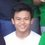# Testing ..

$\begin{equation} x = a_0 + \cfrac{1}{a_1 + \cfrac{1}{a_2 + \cfrac{1}{a_3 + \cfrac{1}{a_4} } } } \end{equation}$

$\alpha, A, \beta, B, \gamma, \Gamma, \pi, \Pi, \phi, \varphi, \Phi$

$\lim_{x \to \infty} \exp(-x) = 0$

$x \equiv a \pmod b$

$k_{n+1} = n^2 + k_n^2 - k_{n-1}$

$\frac{n!}{k!(n-k)!} = \binom{n}{k}$

$\frac{\frac{1}{x}+\frac{1}{y}}{y-z}$

$\frac{ \begin{array}{c}[b]{r} \left( x_1 x_2 \right)\\ \times \left( x'_1 x'_2 \right) \end{array} }{ \left( y_1y_2y_3y_4 \right) }$

$\sqrt[n]{1+x+x^2+x^3+\ldots}$

$\sum_{i=1}^{10} t_i$

$\int_0^\infty \mathrm{e}^{-x}\,\mathrm{d}x$

$\begin{matrix} a & b & c \\ d & e & f \\ g & h & i \end{matrix}$

$A_{m,n} = \begin{pmatrix} a_{1,1} & a_{1,2} & \cdots & a_{1,n} \\ a_{2,1} & a_{2,2} & \cdots & a_{2,n} \\ \vdots & \vdots & \ddots & \vdots \\ a_{m,1} & a_{m,2} & \cdots & a_{m,n} \end{pmatrix}$

$f(n) = \left\{ \begin{array}{l l} n/2 & \quad \text{if \n\ is even}\\ -(n+1)/2 & \quad \text{if \n\ is odd} \end{array} \right.$Note by JohnDonnie Celestre
7 years, 3 months ago

This discussion board is a place to discuss our Daily Challenges and the math and science related to those challenges. Explanations are more than just a solution — they should explain the steps and thinking strategies that you used to obtain the solution. Comments should further the discussion of math and science.

When posting on Brilliant:

• Use the emojis to react to an explanation, whether you're congratulating a job well done , or just really confused .
• Ask specific questions about the challenge or the steps in somebody's explanation. Well-posed questions can add a lot to the discussion, but posting "I don't understand!" doesn't help anyone.
• Try to contribute something new to the discussion, whether it is an extension, generalization or other idea related to the challenge.

MarkdownAppears as
*italics* or _italics_ italics
**bold** or __bold__ bold
- bulleted- list
• bulleted
• list
1. numbered2. list
1. numbered
2. list
Note: you must add a full line of space before and after lists for them to show up correctly
paragraph 1paragraph 2

paragraph 1

paragraph 2

[example link](https://brilliant.org)example link
> This is a quote
This is a quote
    # I indented these lines
# 4 spaces, and now they show
# up as a code block.

print "hello world"
# I indented these lines
# 4 spaces, and now they show
# up as a code block.

print "hello world"
MathAppears as
Remember to wrap math in $$ ... $$ or $ ... $ to ensure proper formatting.
2 \times 3 $2 \times 3$
2^{34} $2^{34}$
a_{i-1} $a_{i-1}$
\frac{2}{3} $\frac{2}{3}$
\sqrt{2} $\sqrt{2}$
\sum_{i=1}^3 $\sum_{i=1}^3$
\sin \theta $\sin \theta$
\boxed{123} $\boxed{123}$

Sort by:

Haha, good idea.

- 7 years, 3 months ago